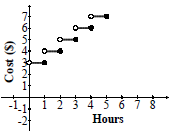### Home > CCA2 > Chapter Ch3 > Lesson 3.1.2 > Problem3-34

3-34.

You decide to park your car in a parking garage that charges $\3.00$ for the first hour and $\1.00$ for each hour (or any part of an hour) after that. Homework Help ✎

1. How much will it cost to park your car for $90$ minutes?

$\4.00$

2. How much will it cost to park your car for $118$ minutes? $119$ minutes?

$\4.00$ for both

3. How much will it cost to park your car for $120$ minutes? $121$ minutes?

$\4.00$ for $120$ minutes, $\5.00$ for $121$ minutes

4. Graph the cost in relation to the length of time your car is parked.5. Is this function continuous?

Can you sketch the graph without lifting your pencil off of the paper?

6. Describe how the graph of this function will change if the parking garage raises their parking rate so that the first hour is now $\5.00$.

Cost is on the $y$-axis. What will happen to the graph is you add $\5.00$ to all of the $y$-values?